﻿ 磨料射流与电火花复合加工技术喷嘴内流场速度分布研究

# 磨料射流与电火花复合加工技术喷嘴内流场速度分布研究Study on Velocity Distribution of Flow Field in Nozzles for the Composite Machining Technology of Abrasive Jet and EDM

Abstract: The composite machining technology (CMT) of abrasive jet (AJ) and electrical discharge machining (EDM) is used to improve the machining quality and efficiency of the workpiece, and the velocity distributions of flow felid of jet fluid in the nozzle and at nozzle exit have important influences on machining process of the workpiece. By establishing the finite element model of the flow field in the nozzle and changing the inlet diameter and inlet velocity of the nozzle, effects of inlet diameter for working fluid, inlet diameter for abrasive, outlet diameter for nozzle, inlet velocity for working fluid, inlet velocity for abrasive, on the velocity distributions of flow fields in nozzle and at nozzle exit, are studied. The results show that the velocities at the nozzle exit increase with the increase of inlet diameters for working fluid and abrasive, however, the distributions of velocity uniformity are worse in these cases; the velocities decrease with the increases of the outlet diameter for the nozzle, and the distributions of velocity uniformity are not much changed; the velocity distributions of flow field in nozzle change a little with the increases of inlet velocity for abrasive at low speeds, however, influences are greater at the high speed cases; for different inlet velocities of working fluid, the velocity distributions of flow field in nozzle have almost the same trend, and the velocities with great values locate mainly below the line between the working fluid inlet and the nozzle outlet. The results in this paper can be used to optimize the internal geometry of the nozzle.

1. 引言

2. 计算模型

2.1. 磨料射流与电火花复合加工技术原理

2.2. 湍流流动的理论基础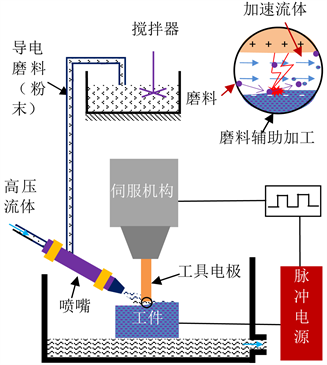Figure 1. Schematic diagram of the CMT of AJ and EDM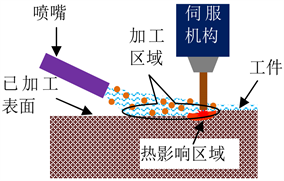Figure 2. The machining area of EDM

$\left\{\begin{array}{l}\frac{\partial \rho }{\partial t}+\frac{\partial \rho {v}_{j}}{\partial {x}_{i}}=0\\ \frac{\partial \rho {v}_{i}}{\partial t}+\frac{\partial \rho {v}_{j}}{\partial {x}_{i}}=-\frac{\partial P}{\partial {x}_{j}}+\frac{\partial }{\partial {x}_{j}}\left[{\mu }_{e}\left(\frac{\partial {v}_{j}}{\partial {x}_{j}}+\frac{\partial {v}_{i}}{\partial {x}_{i}}\right)\right]-\rho {g}_{i}\beta T\\ \frac{\partial \left(\rho k\right)}{\partial {x}_{j}}+\frac{\partial \left(\rho {v}_{j}\right)}{\partial {x}_{j}}=\frac{\partial }{\partial {x}_{i}}\left(\frac{{\mu }_{e}}{{\epsilon }_{k}}\frac{\partial k}{\partial {x}_{j}}\right)+{P}_{k}+{P}_{b}-\rho \epsilon \\ \frac{\partial \rho \epsilon }{\partial t}+\frac{\partial \rho {v}_{k}\epsilon }{\partial {x}_{k}}=\frac{\partial }{\partial {x}_{k}}\left(\frac{{\mu }_{e}}{{\epsilon }_{k}}\frac{\partial \epsilon }{\partial {x}_{j}}\right)+\frac{\epsilon }{k}\left(c{\epsilon }_{1}Pk-\rho c{\epsilon }_{2}\epsilon \right)+c{\epsilon }_{3}\frac{\epsilon }{k}{P}_{k}\end{array}$ (1)

2.3. 喷嘴内流场有限元计算模型

3. 结果与讨论

3.1. 喷嘴内部流场的速度分布Figure 3. Schematic diagram of the nozzle geometry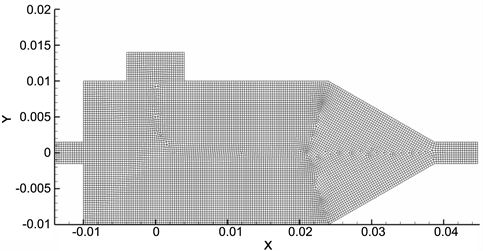Figure 4. The finite element grids of the nozzleTable 1. Geometric dimensioning for the internal structure of the nozzle

3.2. 几何尺寸对出口处流场速度分布的影响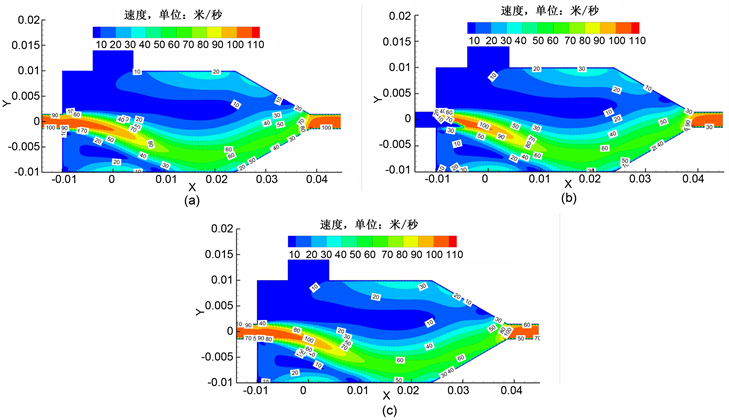Figure 5. The velocity distribution of flow field in the nozzle: (a) The velocity distribution of flow field for the abrasive-working fluid; (b) The velocity distribution of flow field for the abrasive; (c) The velocity distribution of flow field in the nozzle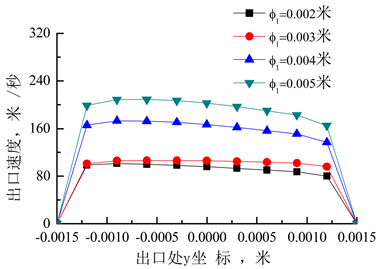Figure 6. Effects of inlet diameter for working fluid on the velocity distribution of the exit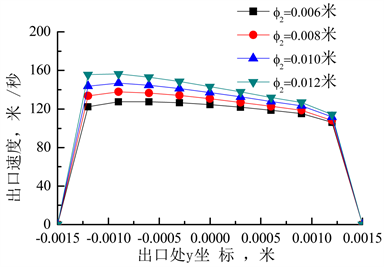Figure 7. Effects of inlet diameter for abrasive on the velocity distribution of the exitFigure 8. Effects of the exit diameter on the velocity distribution of the exit

3.3. 入口速度对喷嘴内部和出口处流场速度分布的影响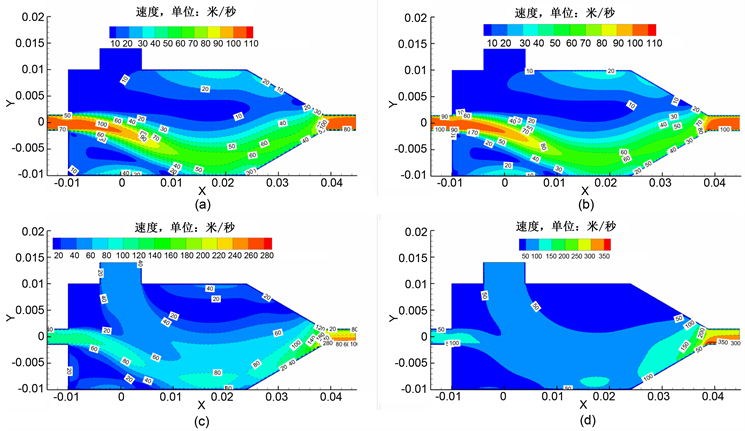Figure 9. Effects of inlet velocity for abrasive on the velocity distribution of the flow field in the nozzle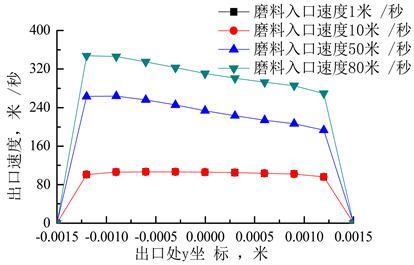Figure 10. Effect of inlet velocity for abrasive on velocity distribution of nozzle outlet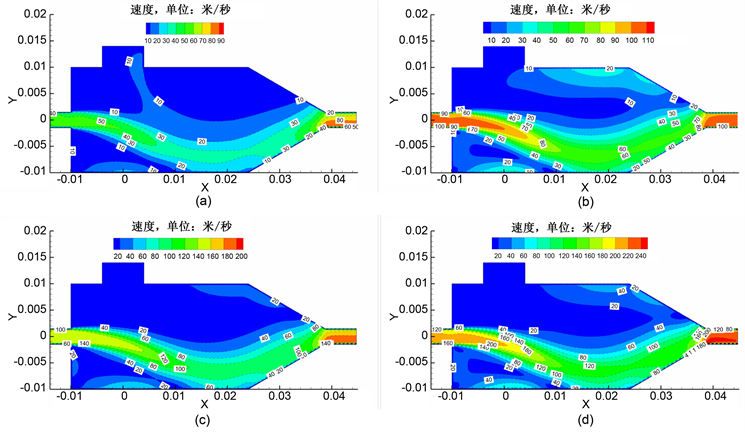Figure 11. Effects of the inlet velocity for working fluid on the velocity distribution of the flow field in the nozzle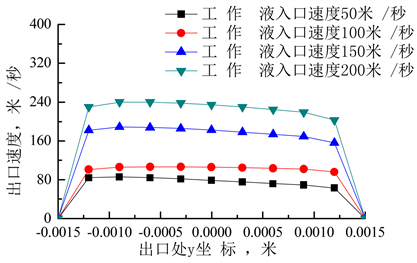Figure 12. Effect of inlet velocity for working fluid on velocity distribution of nozzle outlet

4. 结论

1) 在相同磨料入口速度和工作液入口速度情况下，随着工作液入口尺寸和磨料入口尺寸的增加，喷嘴出口处速度值都会增加，但速度分布均匀性却相应变差；喷嘴出口尺寸的增加，会导致喷嘴出口处速度值的降低，但速度分布均匀性变化不大。

2) 低速时，磨料入口速度增加，对喷嘴内部流场的速度分布状况影响不大；对于不同的工作液入口速度，喷嘴内部流场的速度分布大致一致，速度较大部分主要分布在工作液入口与喷嘴出口连线下部区域。磨料入口速度和工作液入口速度增加，都会导致喷嘴内部流场相应位置流速的增加。

3) 喷嘴几何尺寸、入口速度都会对喷嘴内部流场的速度分布以及喷嘴出口处速度分布产生影响。

4) 在磨料射流与电火花复合加工过程中，针对不同的入射速度，应该设计出具有不同结构尺寸的喷嘴。

 Klocke, F., Zeis, M., Klink, A. and Veselovac, D. (2013) Technological and Economical Comparison of Roughing Strategies via Milling, Sinking-EDM, Wire-EDM and ECM for Titanium- and Nickel-Based Blisks. CIRP Journal of Manufacturing Science and Tech-nology, 6, 198-203.
https://doi.org/10.1016/j.cirpj.2013.02.008

 Yu, Z.Y., Zhang, Y., Li, J., Luan, F. and Guo, D. (2009) High Aspect Ratio Micro-Hole Drilling Aided with Ultrasonic Vibration and Planetary Movement of Electrode by Micro-EDM. CIRP Annals-Manufacturing Technology, 58, 213-216.
https://doi.org/10.1016/j.cirp.2009.03.111

 Lin, Y.C. and Lee, H.S. (2008) Machining Characteristics of Magnetic Force-Assisted EDM. International Journal of Machine Tools and Manufacture, 48, 1179-1186.
https://doi.org/10.1016/j.ijmachtools.2008.04.004

 Joshi, S., Govindan, P., Malshe, A. and Pajurkar, K. (2011) Experimental Characterization of Dry EDM Performed in a Pulsating Magnetic Field. CIRP Annals—Manufacturing Technology, 60, 239-242.
https://doi.org/10.1016/j.cirp.2011.03.114

 Jain, V.K. and Mote, R.G. (2005) On the Temperature and Specific Energy during Electro Discharge Diamond Grinding. International Journal of Advanced Manufacturing Technology, 26, 56-67.
https://doi.org/10.1007/s00170-003-1983-5

 Patel, D. and Tandon, P. (2015) Experimental Investigations of Thermally En-hanced Abrasive Water Jet Machining of Hard-to-Machine Metals. CIRP Journal of Manufacturing Science and Technology, 10, 92-101.
https://doi.org/10.1016/j.cirpj.2015.04.002

 张成光, 张勇, 张飞虎, 栾殿荣. 磨料水射流加工去除模型研究[J]. 机械工程学报, 2015, 51(7): 188-196.

 侯荣国. 磨料水射流切割性能和喷嘴内外流场的仿真研究[D]: [硕士学位论文]. 济南: 山东大学, 2006.

 沈娟. 高压水射流喷嘴的设计及其结构优化[D]: [硕士学位论文]. 苏州: 苏州大学, 2014.

 康磊. 高压磨料水射流喷嘴几何形状与性能研究[D]: [硕士学位论文]. 秦皇岛: 燕山大学, 2015.

 刘国勇, 王宽宽, 陈欣欣, 董栗明, 朱冬梅, 张少军. 后混合磨料水射流除鳞喷嘴内部流场数值模拟[J]. 工程科学学报, 2015, 37(s1): 29-34.

 Long, X., Ruan, X., Liu, Q., Chen, Z. and Xue, S. (2017) Numerical Investigation on the Internal Flow and the Particle Movement in the Abrasive Waterjet Nozzle. Powder Technology, 314, 635-640.
https://doi.org/10.1016/j.powtec.2016.09.089

 Nie, B., Wang, H., Li, L., Zhang, J., Yang, H., Liu, Z., Wang, L. and Li, H. (2011) Numerical Investigation of the Flow Field Inside and Outside High-Pressure Abrasive Waterjet Nozzle. 1st International Sym-posium on Mine Safety Science and Engineering, 26-29 October 2011, Beijing, 48-55.

Top